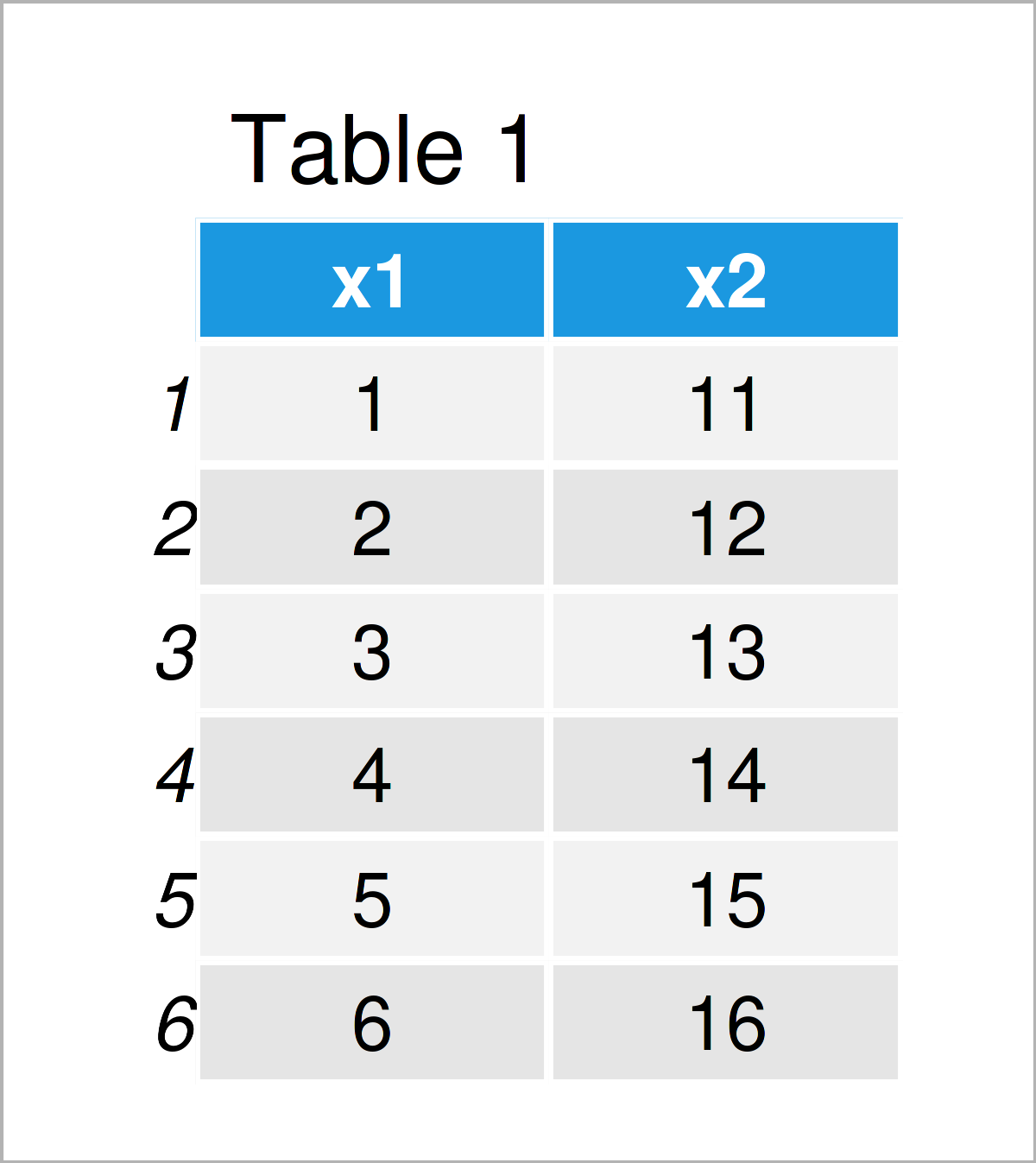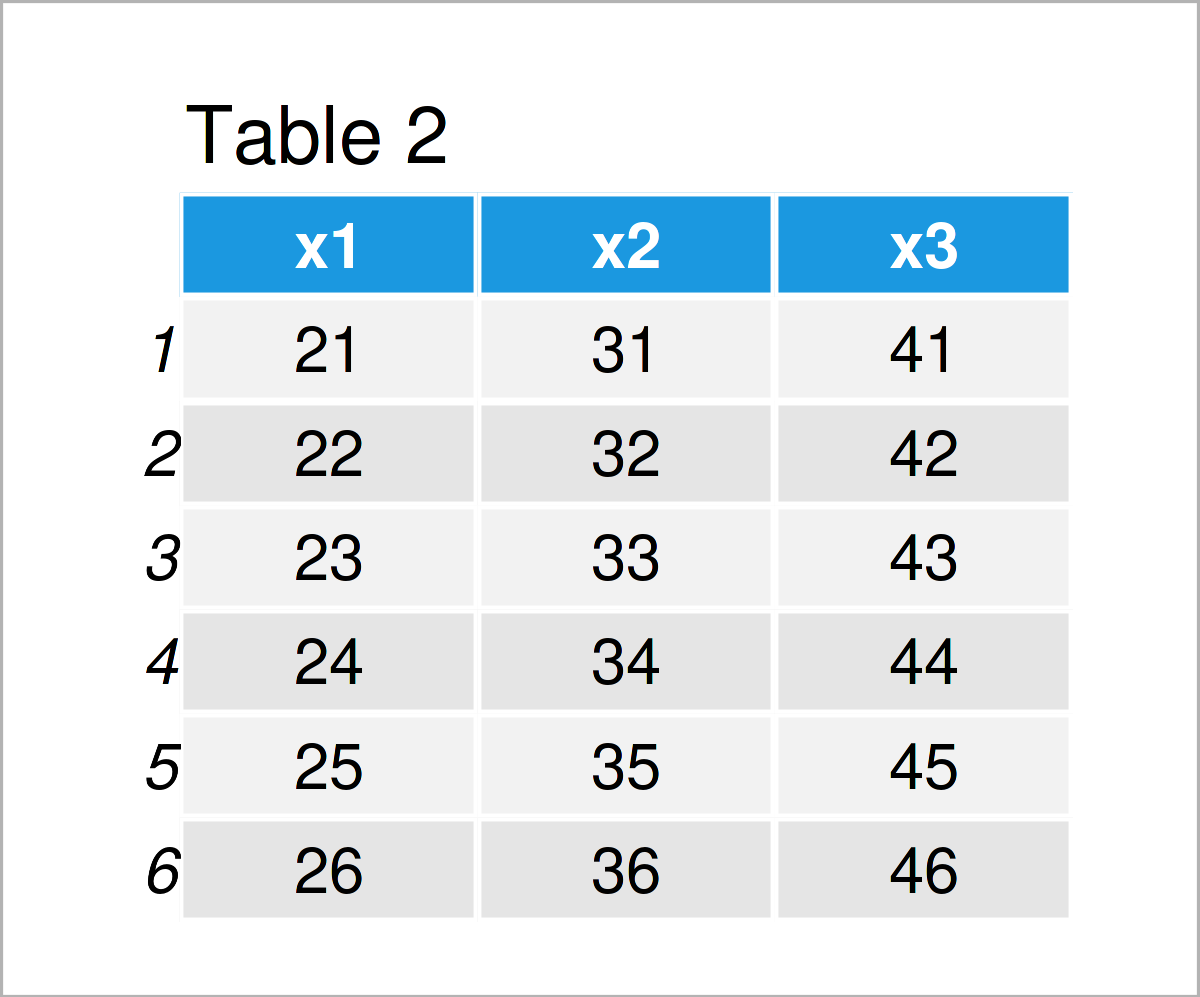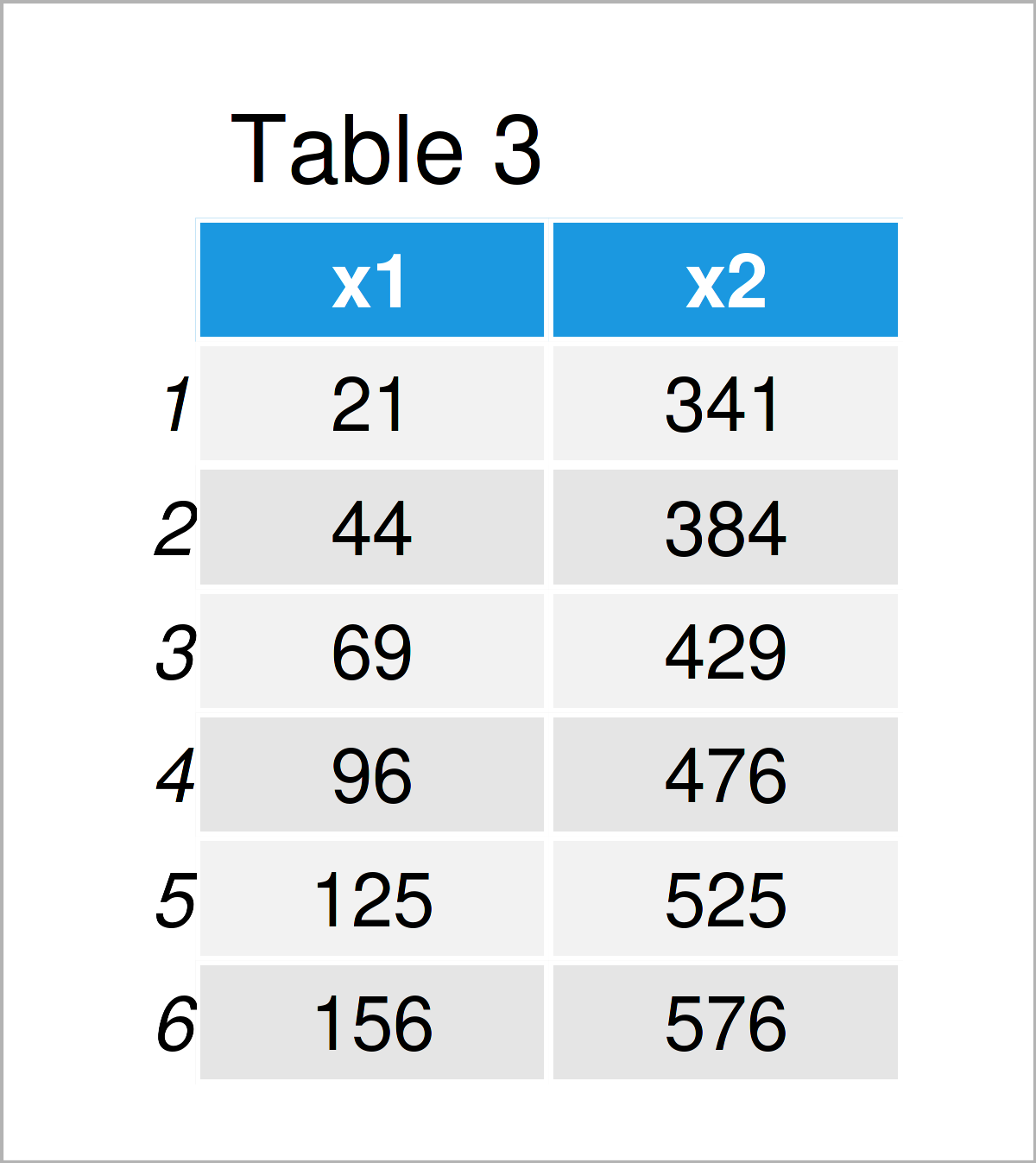# R Error in Ops.data.frame – only defined for equally-sized data frames (2 Examples)

In this tutorial, I’ll show how to reproduce and fix the “Error in Ops.data.frame() : only defined for equally-sized data frames” in R programming.

The article contains two examples for the handling of the “Error in Ops.data.frame() : only defined for equally-sized data frames”. More precisely, the article consists of this content:

Let’s just jump right in.

## Creation of Example Data

The first step is to create some data frames that we can use in the examples later on:

```data1 <- data.frame(x1 = 1:6,                 # Create first data frame
x2 = 11:16)
data1                                         # Print first data frame``````data2 <- data.frame(x1 = 21:26,               # Create second data frame
x2 = 31:36,
x3 = 41:46)
data2                                         # Print second data frame```The output of the previous code is illustrated in Tables 1 and 2 – We have created two different data frames. Note that the first data frame contains two columns, and the second data frame contains three columns.

## Example 1: Reproduce the Error in Ops.data.frame() : only defined for equally-sized data frames

Example 1 demonstrates how to replicate the error message “Error in Ops.data.frame() : only defined for equally-sized data frames”.

Let’s assume that we want to multiply the values of the two data frames data1 and data2. Then, we might try to use the R code below:

```data3 <- data1 * data2                        # Try to multiply data frames
# Error in Ops.data.frame(data, data2) :
#   '*' only defined for equally-sized data frames```

However, the RStudio console returns the “Error in Ops.data.frame() : only defined for equally-sized data frames”.

The reason for this is that our two data frames have a different dimension, i.e. the second data frame has one column more than the first data frame.

This error message often occurs when we are subsetting our data sets, or when we want to remove certain rows for a certain calculation. This can easily lead to data sets that do not have an equal structure anymore.

Let’s fix this!

## Example 2: Fix the Error in Ops.data.frame() : only defined for equally-sized data frames

In Example 2, I’ll explain how to deal with the “Error in Ops.data.frame() : only defined for equally-sized data frames”.

One way to fix this error is the harmonization of the structures of our data sets.

In this specific example, I’m removing all columns from the second data frame that are not contained in the first data set. Note that this depends strongly on your data and what you want to do with your data!

```data4 <- data1 * data2[ , colnames(data1)]    # Properly multiply data frames
data4                                         # Print output```As shown in Table 3, we have created a new data frame by executing the previous R syntax. No errors occurred anymore.

## Video & Further Resources

If you need more info on the content of this article, you could have a look at the following video which I have published on the Statistics Globe YouTube channel. I’m explaining the R syntax of this article in the video.

In addition to the video, you might want to read the related articles on my website. I have released several tutorials already.

In this article you have learned how to handle the “Error in Ops.data.frame() : only defined for equally-sized data frames” in R. Please let me know in the comments section, if you have any further comments or questions. In addition, don’t forget to subscribe to my email newsletter in order to get regular updates on new tutorials.

Subscribe to the Statistics Globe Newsletter

•Snowman
September 24, 2022 6:53 pm

Hi, thanks so much for the tutorial and all the other material you provide! I keep running into this problem, but to me it seems in a different way than described above. I’m trying to write a function with values from various columns on the right-hand side of the equation, such as:
l = alpha – beta*df[i,df\$Column1] – gamma*df[i,df\$Column2]
The columns are of equal length, so I don’t understand what the problem is. Could you please explain what is/could be going wrong?

•September 30, 2022 5:09 pm

Hi Snowman,

Thank you so much for the kind feedback!

Regarding your question, could you please illustrate the structure of your data in some more detail? What is returned when you execute the following R code:

```head(df)
str(df)```

Regards,
Joachim